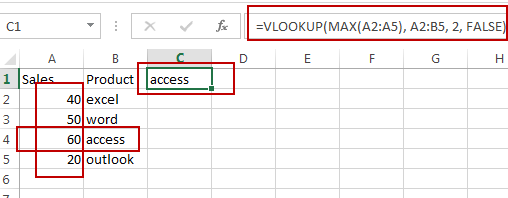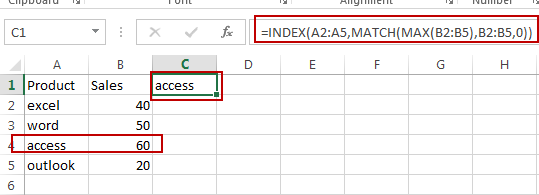# How to Find the Largest Value in a Column and Return the Adjacent Cell Value in Excel

This post will guide you how to find the largest value in a column and then return the adjacent cell value with a formula in Excel. How do I find the max value and return the cell value from the left adjacent column in Excel.

## Find the Largest Value and Return the Adjacent Cell Value

Assuming that you have a list of data in range A1:B5, and you want to find the largest value from the Sales column and then return the adjacent cell value in product column. How to do it. You can use a formula based on the VLOOKUP function and the MAX function to achieve the result. Like this:

`=VLOOKUP(MAX(A2:A5), A2:B5, 2, FALSE)`

Type this formula into a blank cell and press Enter key on your keyboard.You should see that the adjacent cell value is extracted.

Note:  the A2:A5 is the date range that you want to find the largest value. And the MAX function will return the largest value in a given range of cells.

The A2:B5 is the data range that you want to use in the VLOOKUP function.  And the Number 2 is the column number that the matched value is returned, it should be the product column number.

## Find the Max Value and Return Value from Left Adjacent Cell

If you want to find the max value in Sales column and return value from the left adjacent cell in your worksheet, you need to use the INDEX function to create a formula in combination with the MATCH function and the MAX function. Like this:

`=INDEX(A2:A5,MATCH(MAX(B2:B5),B2:B5,0))`

Type this formula into a blank cell and press Enter key on your keyboard.### Related Functions

• Excel INDEX function
The Excel INDEX function returns a value from a table based on the index (row number and column number)The INDEX function is a build-in function in Microsoft Excel and it is categorized as a Lookup and Reference Function.The syntax of the INDEX function is as below:= INDEX (array, row_num,[column_num])…
• Excel MATCH  function
The Excel MATCH function search a value in an array and returns the position of that item.The MATCH function is a build-in function in Microsoft Excel and it is categorized as a Lookup and Reference Function.The syntax of the MATCH function is as below:= MATCH  (lookup_value, lookup_array, [match_type])….
• Excel MAX function
The Excel MAX function returns the largest numeric value from the numbers that you provided. Or returns the largest value in the array.= MAX(num1,[num2,…numn])…
• Excel VLOOKUP function
The Excel VLOOKUP function lookup a value in the first column of the table and return the value in the same row based on index_num position.The syntax of the VLOOKUP function is as below:= VLOOKUP (lookup_value, table_array, column_index_num,[range_lookup])….

Sidebar